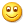## 小tip: 了解LinearRGB和sRGB以及使用JS相互转换

### 一、了解LinearRGB和sRGB

LinearRGB顾名思义就是线性RGB颜色。sRGB世界中，RGB色值范围是0~255，中灰色就是`127`，使用0~1范围表示就是`0.5`，但是，转换成LinearRGB就是`0.214`

### 二、LinearRGB和sRGB使用JS相互转换

LinearRGB转换为sRGB：

```var linear = xxx;  // xxx是0-1的数值
var s;
if (linear <= 0.0031308) {
s = linear * 12.92;
} else {
s = 1.055 * Math.pow(linear, 1.0/2.4) - 0.055;
}```

sRGB转换为LinearRGB：

```var s = xxx;    // xxx是0-1的数值
var linear;
if (s <= 0.04045) {
linear = s / 12.92;
} else {
linear = Math.pow((s + 0.055) / 1.055, 2.4);
}
```

`s = 1.055 * Math.pow(0.5, 1.0/2.4) - 0.055 = 0.7353569830524495`

`Math.floor(0.7353569830524495 * 256) = 188`

### 三、结束语（本篇完）

﻿
1. yty说道：

可太受用了！！！万分感谢分享！但是还有个疑问，比如相机拍摄的图像它是srgb吗，如果不是的话，把他放在srgb显示屏上，会自动转换颜色吗？可以邮箱回我吗？

2. 哦了说道：

asd